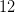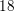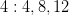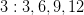SSAT Upper Level Math : Lowest Common Denominator

Example Questions

← Previous 1

Example Question #1 : How To Find The Lowest / Least Common Denominator

Find the least common denominator for the fractions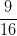and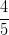.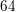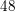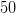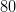Explanation:

To find the least common denominator, list out the multiples for each denominator then choose the lowest one that appears in both sets.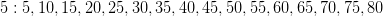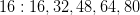Example Question #2 : How To Find The Lowest / Least Common Denominator

Find the least common denominator for the fractions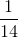and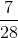.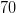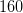Explanation:

To find the least common denominator, list out the multiples for each denominator then choose the lowest one that appears in both sets.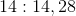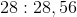Example Question #3 : How To Find The Lowest / Least Common Denominator

What is the least common denominator for the fractionsand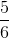?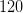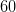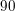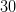Explanation:

To find the least common denominator, list out the multiples for each denominator then choose the lowest one that is in both sets.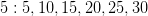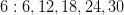Example Question #4 : How To Find The Lowest / Least Common Denominator

Find the least common denominator for the fractionsand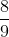.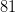Explanation:

To find the least common denominator, list out the multiples for each denominator then choose the lowest one that appears in both sets.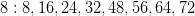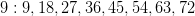Example Question #5 : How To Find The Lowest / Least Common Denominator

Find the least common denominator for the fractions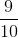and.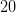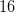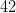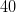Explanation:

To find the least common denominator, list out the multiples for each denominator then choose the lowest one that appears in both sets.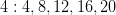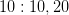Example Question #6 : How To Find The Lowest / Least Common Denominator

Find the least common denominator for the fractions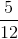and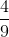.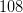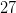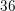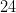Explanation:

To find the least common denominator, list out the multiples for each denominator then choose the lowest one that appears in both sets.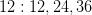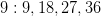Example Question #7 : How To Find The Lowest / Least Common Denominator

Find the least common denominator for the fractionsand.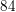Explanation:

To find the least common denominator, list out the multiples for each denominator then choose the lowest one that appears in both sets.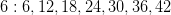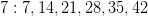Example Question #8 : How To Find The Lowest / Least Common Denominator

Find the least common denominator for the fractions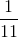and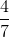.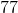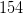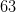Explanation:

To find the least common denominator, list out the multiples for each denominator then choose the lowest one that appears in both sets.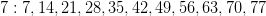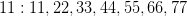Example Question #9 : How To Find The Lowest / Least Common Denominator

Find the least common denominator for the fractionsand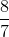.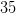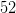Explanation:

To find the least common denominator, list out the multiples for each denominator then choose the lowest one that appears in both sets.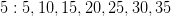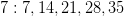Example Question #10 : How To Find The Lowest / Least Common Denominator

Find the least common denominator for the fractions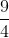and.